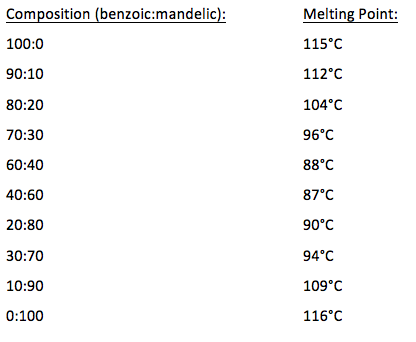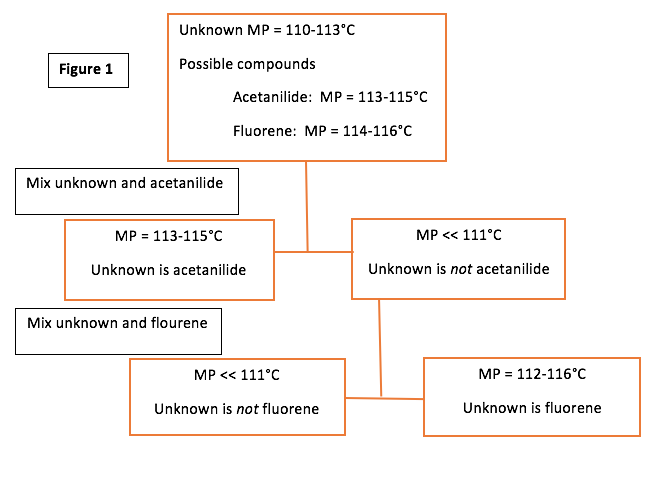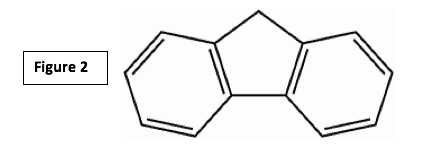# Measuring the Melting Points of Compounds and Mixtures

1. Overview

Our unknown for this experiment was #4.

The experiment had two different sections with different goals in each.  In the first part, we measured the melting points of benzoic acid and mandelic acid, as well as different mixtures of these.  The mixtures were: 80% benzoic with 20% mandelic, 60% benzoic with 40% mandelic, 40% benzoic with 60% mandelic, and 20% benzoic with 80% mandelic.  This was done to obtain information about the eutectic composition and temperature.  In the second section of the experiment, we measured the melting point of an unknown chemical, then added this to two known chemicals with similar known melting points.  This was done to determine the identity of the unknown.

1. Main Body

After measuring the various sample mixtures of benzoic acid and mandelic acid, and calculating the results, I was able to determine that the eutectic temperature is 80° C, and the eutectic composition is 50% each of benzoic acid and mandelic acid.

From the graph made in the post-lab questions, the following information about the mixtures can be determined:If we were to look at a mixture of 80:20 benzoic acid-mandelic acid (for simplicity in explaining, I will use 80µ of benzoic acid, and 20 µg of mandelic acid), the following would happen:  The mixture will begin to melt at the eutectic temperature of 80°C.  At this temperature, the eutectic composition is 50% each of benzoic acid and mandelic acid.  The temperature remains there until all 20µg of mandelic acid melts, and 20µg of benzoic acid melts.  The remaining 60µg of benzoic acid melts over the range of 80-121°C.  At 121°C, the last traces of benzoic acid melt.

Now, to look at what we learned from the second section of the experiment. To identify the unknown, we followed the procedure of the flow chart in Figure 1.From this information, we determined that fluorene is our unknown because our mixture of acetanilide and unknown melted at 88°C, while our mixture of fluorine and unknown melted at 112°C.

It is always very helpful to think about and try to figure out the structural formulas of the chemicals we were working with.  The structural formula for our unknown, fluorine, is shown in Figure 2.Also, we were told to simultaneously measure the melting points of each mixture and the pure unknown.  The reason for this would be so that we could easily see if the unknown and the mixture were melting similarly (at the same rate).

1. Analysis

For the first section, our results gave us a good basis to say that the eutectic temperature is 80°C and the eutectic composition is 50% each of the benzoic and mandelic acids.  We were only able to determine this information because of the very relevant information we found in our measurements of the melting points of the various mixtures of benzoic acid and mandelic acid.

In the second section of the experiment, we determined our unknown to be fluroene.  This was very obviously the choice because the first mixture (unknown and acetanilide) had a much lower melting point (88°C) than the known melting point of pure acetanilide (113-115°C).  The unknown on its own had a melting point of 112°C, and the mixture of unknown and fluorene also had a melting point of 112°C.  Pure fluorene has a known melting point of 114-116°C.  These numbers are very close, unlike the melting point of the mixture of acetanilide and unknown compared to pure acetanilide.

When calculating the amount of benzoic acid that would melt along with the 20µg of mandelic acid at 80°C in an 80:20 benzoic acid-mandelic acid mixture, I used the following equation:

20µg X 50/50 = 20µg

1. Summary/Conclusion

This experiment allowed us to determine the eutectic temperature and composition of a benzoic acid-mandelic acid mixture by measuring the melting points of these pure substances, as well as various compositions of them.  We were also able to use melting points to determine an unknown substance (#4) by obtaining the melting point of the unknown and then mixing it with two substances with known similar melting points and finding out which melting point was not affected by the addition of the unknown.

1. Reflections

In this lab, I learned how to fill/pack capillary tubes, and how to use the MelTemp equipment.  I had previously known that melting points can be a way to determine an unknown, but had not previously practiced it myself.  I was able to do this in this lab as well.  If I was to repeat this procedure, I would probably start slowing the rising temperature a little lower, so as to obtain the most accurate melting points I possibly could.

• Share